# Class 10 Maths Chapter 8: Introduction to Trigonometry Ex 8.1

In this page we have NCERT Solutions for Class 10 Maths Chapter 8: Introduction to Trigonometry for EXERCISE 8.1 . Hope you like them and do not forget to like , social_share and comment at the end of the page.
Question 1
In Δ ABC, right-angled at B, AB = 24 cm, BC = 7 cm. Determine:
(i) Sin A, cos A
(ii) Sin C, cos C
Solution
In Δ ABC, right-angled at B ,using Pythagoras theorem we have
AC= AB2 +BC2  = 576 + 49 = 625
Or AC=25 ( taking positive value only)
Now
(i) In a right angle triangle ABC where B=90° ,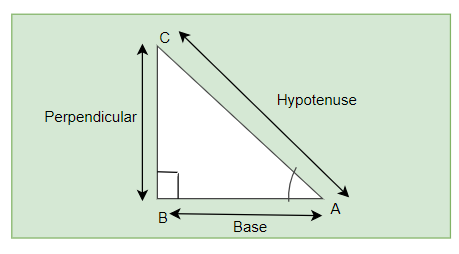Sin A = BC/AC = 7/25
CosA = AB/AC = 24/25
(ii)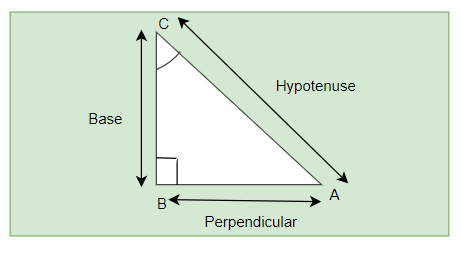Sin C = AB/AC = 24/25
Cos C = BC/AC =7/25

Question 2
In below find tan P – cot R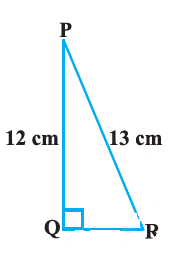Solution
Again consider the above figure . Now by Pythagoras theorem
PQ2 + QR2  =PR2
QR=5
Now
tan P = Perp/Base = 5/12
Cot R = Base/Perm = 5/12
So tan P – cot R=0

Question 3. If $sin A= \frac{3}{4}$. Calculate cos A and tan A.
Solution
Given $sin A= \frac{3}{4}$
Or P/H=3/4
Let  P=3k and H=4kNow By Pythagoras theorem
P2 + B2 =H2
9k2 + B2 =16k2
Or  $B =+ k \sqrt 7$
Now $\cos A = {B \over H} = {{\sqrt 7 } \over 4}$
Now $\tan A = {{\sin A} \over {\cos A}} = {3 \over {\sqrt 7 }}$
Question 4
Given 15 cot A = 8, find sin A and sec A.

Solution 4
$Cot A =\frac{8}{15}$
Or
${B \over P} = {8 \over {15}}$
Let B=8K and P=15kSo in a right angle triangle with angle A
P2 + B2 =H2
Or H=17K
Sin A = P/H = 15/17
Sec A = H/B = 17/8

Question 5
Given sec θ =13/12. Calculate all other trigonometric ratios.
Solution 5
Given sec θ=13/12
Or
H/B=13/12
let H=13K ,B=12K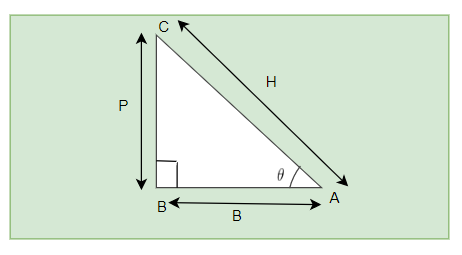So in a right angle triangle with angle A
P2 + B2 =H2
P=5k
sin θ = P/H = 5/13
Cos θ = B/H = 12/13
Tan θ = P/B = 5/12
Cosec θ= 1/sin θ = 13/5
cot θ = 1/tan θ = 12/5

Question 6
If ∠ A and ∠ B are acute angles such that cos A = cos B, then show that ∠ A = ∠ B.
Solution 6
In a triangle
Cos A =cos B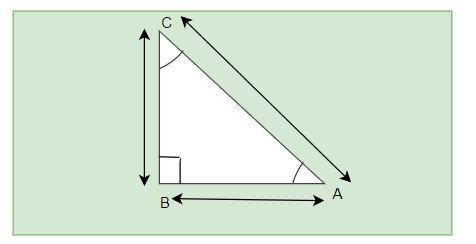AC/AB= BC/AB
⇒AC=BC
⇒Angle A and Angle B

Question 7
If cot θ =7/8 evaluate
(i) ${{\left( {1 + \sin\theta } \right)\left( {1 - \sin\theta } \right)} \over {\left( {1 + \cos\theta } \right)\left( {1 - \cos\theta } \right)}}$
(ii) ${\cot ^2}\theta$
Solution
Given
Given cot θ=7/8
Or
B/P=7/8
let B=7K ,P=8KSo in a right angle triangle with angle θ
P2 + B2 =H2
$H = k\sqrt {113}$
$\sin \theta = {P \over H} = {8 \over {\sqrt {113} }}$
$\cos \theta = {B \over H} = {7 \over {\sqrt {113} }}$
(i) ${{\left( {1 + sin\theta } \right)\left( {1 - sin\theta } \right)} \over {\left( {1 + cos\theta } \right)\left( {1 - cos\theta } \right)}}$
$= {{1 - \sin^2 \theta } \over {1 - \cos^2 \theta }}$
${{1 - {{64} \over {113}}} \over {1 - {{49} \over {113}}}} = {{49} \over {64}}$
(ii)Cot2 θ=(cot θ)2= 49/64

Question 8
If 3 cot A = 4, check whether below is true or not
${{1 - \tan^2 A} \over {1 + \tan^2 A}} = \cos^2 A - \sin^2 A$
Solution 8
Given
Cot A=4/3
Or
B/P=4/3
Let B=4k and P=3k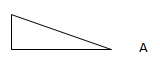So in a right angle triangle with angle A
P2 + B2 =H2
H=5k
Now tan A=1/cot A=3/4
Cos A=B/H=4/5
Sin A=P/H=3/5
Let us take the LHS
${{1 - ta{n^2}A} \over {1 + ta{n^2}A}} = {{1 - {{\left( {{3 \over 4}} \right)}^2}} \over {1 + {{\left( {{3 \over 4}} \right)}^2}}} = {7 \over {25}}$
RHS=cos2 A -  sin2A= 7/25
So LHS=RHS,so the statement is true

Question 9
In triangle ABC, right-angled at B, if $\tan A = {1 \over {\sqrt 3 }}$
Find the value of:
(i) sin A cos C + cos A sin C
(ii) cos A cos C – sin A sin C
Solution
$\tan A = {1 \over {\sqrt 3 }}$
${P \over B} = {1 \over {\sqrt 3 }}$
Let $P = k$ and $B = k\sqrt 3$
Now by Pythagoras theorem
P2 + B2 =H2
H=2k
(i)
$\sin A \cos C + \cos A \sin C = \left( {{P \over H}} \right)\left( {{B \over H}} \right) + \left( {{B \over H}} \right)\left( {{P \over H}} \right)$
$= \left( {{{BC} \over {AC}}} \right)\left( {{{BC} \over {AC}}} \right) + \left( {{{AB} \over {AC}}} \right)\left( {{{AB} \over {AC}}} \right)$
$= {{{k^2}} \over {4{k^2}}} + {{3{k^2}} \over {4{k^2}}} = 1$
(ii)
$\cos A \cos C - \sin A \sin C = \left( {{P \over H}} \right)\left( {{P \over H}} \right) + \left( {{B \over H}} \right)\left( {{B \over H}} \right)$
$= \left( {{{BC} \over {AC}}} \right)\left( {{{AB} \over {AC}}} \right) - \left( {{{AB} \over {AC}}} \right)\left( {{{BC} \over {AC}}} \right)$
$=0$

Question 10
In Δ PQR, right-angled at Q, PR + QR = 25 cm and PQ = 5 cm. Determine the values of
sin P, cos P and tan P.
Solution
Let QR=x andPR=y
Then x+y=25
y=25-x
Now by Pythagorus theorem
x2  + 25= y2
x2  + 25==(25-x)2
Solving it ,we get
X=12 cm
Then y=25-12=13 cm
Now Sin P= 12/13
Cos P=5/13
Tan P=12/5

Question 11
(i) The value of tan A is always less than 1.
(ii) sec A =12/5 for some value of angle A.
(iii) cos A is the abbreviation used for the cosecant of angle A.
(iv) cot A is the product of cot and A.
(v) sin θ =4/3 for some angle
Solution
1. False. The value of  tan A increase from 0 to ∞.
2. True. The value of sec A increase from 1 to ∞.
3. False .Cos A is the abbreviation used for the cosine of angle A
4. False .cot A is one symbol. We cannot separate it
5. False. The value of sin θ always lies between 0 and 0 and 4/3 > 1Go back to Class 10 Main Page using below links

### Practice Question

Question 1 What is $1 - \sqrt {3}$ ?
A) Non terminating repeating
B) Non terminating non repeating
C) Terminating
D) None of the above
Question 2 The volume of the largest right circular cone that can be cut out from a cube of edge 4.2 cm is?
A) 19.4 cm3
B) 12 cm3
C) 78.6 cm3
D) 58.2 cm3
Question 3 The sum of the first three terms of an AP is 33. If the product of the first and the third term exceeds the second term by 29, the AP is ?
A) 2 ,21,11
B) 1,10,19
C) -1 ,8,17
D) 2 ,11,20